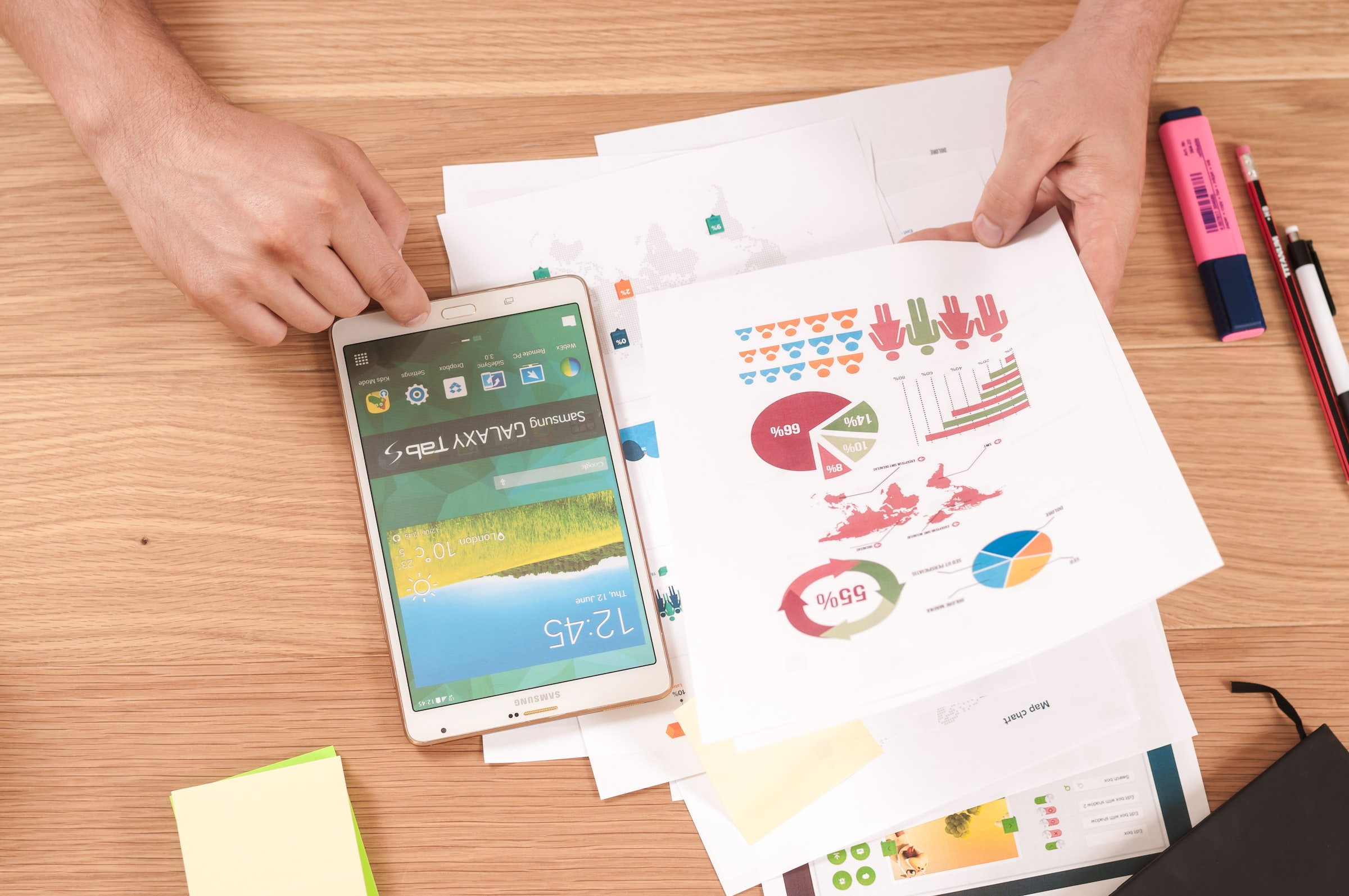# Linear and declining-balance depreciation

Share now!## Fixed assets such as machines, vehicles, furniture or IT equipment that are used over several years are capitalised in the balance sheet at the time of purchase. In the course of time, the value of these assets decreases. The reduction in value of tangible assets is recognised as an expense through depreciation. There are two different methods of depreciating an asset: straight-line and declining balance.

### Linear depreciation

With straight-line depreciation, the asset is depreciated at the same value each year. The acquisition value is divided by the expected service life, and the resulting amount is depreciated.

Example

We buy a machine for CHF 10'000. We assume that this machine will be used for 5 years. We therefore divide CHF 10,000 by the expected life of 5 years and get CHF 2,000. We therefore depreciate the machine annually by CHF 2,000, so that it has a book value of 0 after 5 years.

### Declining-balance depreciation

With declining balance depreciation, a percentage of the residual book value is always depreciated. Depreciation thus decreases annually until the entire residual value is written off at the end of the useful life.

Example

We buy a vehicle for CHF 50,000 and want to use it for 5 years. We decide to depreciate the vehicle on a declining balance basis at a rate of 40% (40% of 50,000 = 20,000). After the first year, the vehicle has a residual book value of CHF 30'000 (50'000-20'000=30'000). In the next year, 40% of the 30,000 is depreciated, i.e., CHF 12,000, resulting in a residual book value of CHF 18,000.Competent specialistsTransparent prices